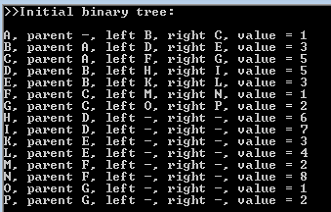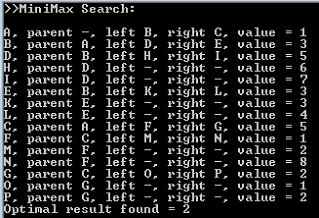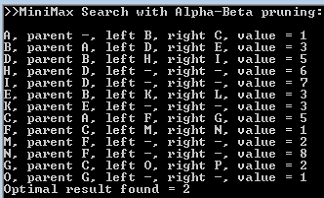# Alpha Beta Pruning Example: Part 3

Create a binary tree of 15 nodes which use the following names:

```                [A]
/        \
[B]             [C]
/     \         /     \
[D]    [E]      [F]    [G]
/  \   /  \     /  \   /   \
[H] [I] [J] [K] [L] [M] [N] [O]
```

with the nodes holding the following values:

```              
/         \
             
/      \        /     \
               
/  \    /   \   /   \   /   \
       
```
• The binary tree must be created automatically from an array containing the values: {1,3,5,5,3,1,2,6,7,3,4,2,8,1,2}.
• Display the tree in console window showing the following information: node name, parent node name, children node names, and the value stored in each node. Hyphenate the non-existing parent/children nodes.

Imagine that this tree represents a set of possible turns between two players playing a game. The turn corresponding to node A belongs to player who wants to minimize the results; the opposing player wants to maximize them.

• Perform a MiniMax search of binary tree, and display the order nodes were traversed, as well as the optimal leaf node value found.
• Enhance your MiniMax search algorithm so that it includes Alpha-Beta pruning. Repeat the search, and again display the results. Are there any visible differences? Write down your observations.

SOLUTION:

In the previous samples, we have learned how to create and traverse a binary tree. Now, we are going to show the MiniMax search (as well as its Alpha-Beta pruning extension) using this very tree. First of all, we will need to create a tree structure consisting from nodes. Each node can have a parent node, and (since its Binary tree) two children nodes: left and right. Each node also has a name, and a value it carries. Node class will look as following:

```public class Node
{
public Node left { get; set; }
public Node right { get; set; }
public Node parent { get; set; }
public string name { get; set; }
public int value { get; set; }

public Node(Node p, string n, int v)
{
parent = p;
name = n;
value = v;
}
}

```

The pre-requisites for creation of our binary tree include a pair of arrays (one housing values and the other names of nodes):

```//Array of binary tree node values:
int[] arr = new int[] { 1,  3, 5,  5, 3, 1, 2,  6, 7, 3, 4, 2, 8, 1, 2 };
//Array of letter markers for discerning the nodes easier:
string[] arr2 = new string[] { "A", "B","C", "D","E","F","G", "H","I","K","L","M","N","O","P" };
```

Some other pre-requisites:

```//Array which will house the number of nodes required:
Node[] tree = new Node[arr.Length];
//Nonexisting parent of the very first node in the tree:
Node parent = null; //We will use this variable to store the parents of subsequent nodes as well
```

We will go through the “tree” array, creating the nodes. To create the node, we need to provide a parent node, name, and numerical value respectively:

```tree[i] = new Node(parent, arr2[i], arr[i]);
```

But we should take into account that the “null” parent is only fitting for the very first “parentless” node “A”. For all subsequent nodes, we need a mechanism that will assign proper parents to the next branches of 2, 4, 8 nodes and so on:

```if (i > 0) parent = tree[(i + (i % 2 == 0 ? 0 : 1) - 2) / 2];
```

Which makes for the following array-filling code:

```for (int i = 0; i < arr.Length; i++) { if (i > 0) parent = tree[(i + (i % 2 == 0 ? 0 : 1) - 2) / 2];
tree[i] = new Node(parent, arr2[i], arr[i]);
}
```

We are using arr.Length and not tree.Length since all of the arrays in this application have same length anyway. At this moment, we should have 15 nodes with “parent” attribute being filled. However, we have no children nodes yet. To fix this, we need to iterate through this array once again:

```//setting children nodes now that the every required node was created
for (int i = 0; i < arr.Length; i++)
{
//the last half of nodes lacks further children nodes, so they are set to null
tree[i].left = (i < arr.Length / 2) ? tree[i + i + 1] : null;
tree[i].right = (i < arr.Length / 2) ? tree[i + i + 2] : null;
}
```

Now we need to display the binary tree (which we will do on node-per-line basis), and for this we will create the appropriate function:

```static void ShowNodeData(Node n)
{
Console.Write("{0}, ", n.name);
if (n.parent != null) Console.Write("parent {0}, ", n.parent.name);
else Console.Write("parent -, ");
if (n.left != null) Console.Write("left {0}, ", n.left.name);
else Console.Write("left -, ");
if (n.right != null) Console.Write("right {0}, ", n.right.name);
else Console.Write("right -, ");
Console.WriteLine("value = {0}", n.value);
}
```

Calling this function from main part of application for every node in our array allows to display data about the whole binary tree:

```//displaying the binary tree
Console.WriteLine(">>Initial binary tree:");
Console.WriteLine();
foreach (Node item in tree)
{
ShowNodeData(item);
}
```

Result:MiniMax search essentially assumes that the different height levels of binary tree correspond to alternating turns of two opposing players, one of which searches to Minimize the outcome, and the other to Maximize it. Each node represents a turn of one of the players, with leaf nodes (the ones having no children nodes) being of major interest to the players. MiniMax search therefore attempts to detect which turn will be most advantageous to the particular player in the long run.

MiniMax search function:

```static int MiniMax(Node n, bool MaximizingPlayer)
{
ShowNodeData(n);

int bestValue;
List<Node> nchildren = new List<Node>();

if (nchildren.Count == 0) bestValue = n.value;
else if (MaximizingPlayer)
{
bestValue = -int.MaxValue;
for (int i = 0; i < nchildren.Count; i++)
{
var childvalue = MiniMax(nchildren[i], false);
bestValue = Math.Max(bestValue, childvalue);
}
}
else
{
bestValue = int.MaxValue;
for (int i = 0; i < nchildren.Count; i++)
{
var childvalue = MiniMax(nchildren[i], true);
bestValue = Math.Min(bestValue, childvalue);
}
}
return bestValue;
}
```

Calling this function:

```Console.WriteLine(">>MiniMax Search:");
Console.WriteLine();
Console.WriteLine("Optimal result found = {0}", MiniMax(tree, false));
```

results in:One downside to MiniMax search is that it traverses the whole tree, which is simply not viable when dealing with large enough trees (such as the ones used in chess and other games). Alpha-Beta pruning acts as a way to improve basic MiniMax algorithm performance, as it allows to skip certain node branches during the search, therefore eliminating them from the traversal.

The basic idea behind Alpha-Beta pruning is the introduction of two more variables: Alpha (max score that’s guaranteed to maximizing player), and Beta (min. score that’s guaranteed to minimizing player). This is useful since “Alpha ≥ Beta” situation on any branch assures that other branches have more beneficial scores for either maximizing or minimizing players, effectively eliminating the current branch from traversal.

MiniMax search function updated with Alpha-Beta pruning:

```static int AlphaBeta(Node n, int alpha, int beta, bool MaximizingPlayer)
{
ShowNodeData(n);

int bestValue;
List<Node> nchildren = new List<Node>();

if (nchildren.Count == 0) bestValue = n.value;
else if (MaximizingPlayer)
{
bestValue = alpha;
for (int i = 0; i < nchildren.Count; i++)
{
var childvalue = AlphaBeta(nchildren[i], bestValue, beta, false);
bestValue = Math.Max(bestValue, childvalue);
if (beta <= bestValue) break ;
}
}
else
{
bestValue = beta;
for (int i = 0; i < nchildren.Count; i++)
{
var childvalue = AlphaBeta(nchildren[i], alpha, bestValue, true);
bestValue = Math.Min(bestValue, childvalue);
if (bestValue <= alpha) break;
}
}
return bestValue;
}
```

Calling this function:

```Console.WriteLine();
Console.WriteLine(">>MiniMax Search with Alpha-Beta pruning:");
Console.WriteLine();
Console.WriteLine("Optimal result found = {0}", AlphaBeta(tree, -int.MaxValue, int.MaxValue, false));
```

results in:Indeed, we can confirm that while achieving the same result value-wise, the Alpha-Beta pruning actually decreases the amount of nodes traversed in order to reach this result, increasing the overall efficiency.

This is the third and the last part in an alpha beta pruning example series created by our programming specialists. Check out the previous one here – https://assignmentshark.com/blog/minimax-alpha-beta-pruning-example-part-2/. Any usage of the information provided on this page without proper references is prohibited. If you need an algorithm that will pass all tests, then you have come to the right place. Describe your assignment, place an order and wait until our experts complete your very own alpha beta pruning example and send it to you. No more worries about doing piles of assignments and meeting deadlines with expert academic helpers from AssignmentShark! We are waiting for your order.

You can also check out minimax algorithm example about tic tac toe game.